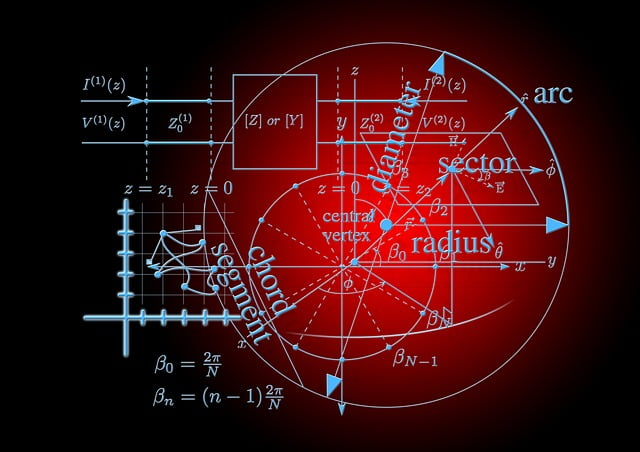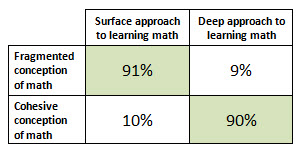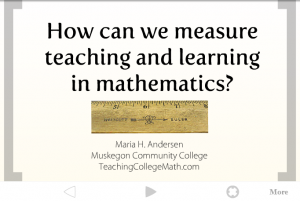Do you ever get the feeling that you’re not making any real progress with your students?  Sure, they pass tests and progress through the courses (well, most of them), but have you ever just had the uneasy feeling that they really don’t get what math is all about?  Suppose you were to ask your students the following question:

Think about the math that you’ve done so far.  What do you think mathematics is?

What do you think they would tell you?

Well, in 1994, a research group from Australia did ask 300 first-year university students this question (Crawford, Gordon, Nicholas, and Prosser).  They identified patterns in the responses, and classified all 300 responses into categories of conceptions in order to explore the relationships between (a) conceptions of mathematics, (b) approaches to learning mathematics, and (c) achievement.

If you want all the gory details about the study, you’ll have to track down the journal article, but let me attempt to summarize their findings.

Students’ conceptions of mathematics fell into one of five categories (Crawford et al., 1994, p. 335):

1. Math is numbers, rules, and formulas.
2. Math is numbers, rules, and formulas which can be applied to solve problems.
3. Math is a complex logical system; a way of thinking.
4. Math is a complex logical system which can be used to solve complex problems.
5. Math is a complex logical system which can be used to solve complex problems and provides new insights used for understanding the world.

The first two categories represent a student view of mathematics that is termed “fragmented” while the last three categories present a more “cohesive” view of mathematics.  Note that the terms fragmented and cohesive are well-used throughout the international body research.  The categories above, as you may have noticed, form a hierarchical list, with each one building on the one above it.

Here’s where this study gets (even more) interesting.  The researchers also asked students about how they studied (changing the language slightly here to “americanize it” a bit):

Think about some math you understood really well. How did you go about studying that?  (It may help you to compare how you studied this with something you feel you didn’t fully understand.) How do you usually go about learning math?

Again, the researchers went through a meticulous process of categorization and came up with five categories (Crawford et al., 1994, p. 337):

1. Learning by rote memorization, with an intention to reproduce knowledge and procedures.
2. Learning by doing lots of examples, with an intention to reproduce knowledge and procedures.
3. Learning by doing lots of examples with an intention of gaining a relational understanding of the theory and concepts.
4. Learning by doing difficult problems, with an intention of gaining a relational understanding of the entire theory, and seeing its relationship with existing knowledge.
5. Learning with the intention of gaining a relational understanding of the theory and looking for situations where the theory will apply.

Again, these five categories were grouped, this time according to intention, into two general categories: reproduction and understanding.  In the first two approaches to learning math, students simply try to reproduce the math using rote memorization and by doing lots of examples.  In the last three categories, students do try to understand the math, by doing examples, by doing difficult problems, and by applying theory.  Other researchers in this community have seen similar results on both general surveys of student learning and on subject-specific surveys and have termed this to be surface approach and deep approach to learning (see Marton, 1988).

Still reading?  Good.  Remember my first question? Do you ever get the feeling that you’re not making any real progress with your students? Let’s answer that now.

Here’s how the conceptions of math and the approaches to learning math correlated in this study (Crawford et al., p. 341):Did you catch that?  Look at how strongly conception and approach correlates.  It’s probably what you’ve always suspected, deep down inside, … but there’s the cold, hard, proof.

Of course, all this is not so meaningful unless there’s a correlation with achievement.  At the end of their first year, the students’ final exam scores were compared to the conceptions and approaches to mathematics (again, for technical details, get the article). The researchers made two statistically significant findings:

1. Students with a cohesive conception of math tended to achieve at a higher level (p < .05).
2. Students with a deep approach to learning math tended to achieve at a higher level (p < .01).

Okay, so where does this leave us?  Well, we don’t have causation, only correlation (at least, that’s all we have from the 1994 study).  However, Crawford, Gordon, Nicholas, and Prosser were nice enough to use their research to develop a survey inventory that we can use to measure students’ conceptions of mathematics (1998).  The 19-item inventory (5-point Likert scales) has been thoroughly tested for validity and reliability, and can be found in their 1998 paper, University mathematics students’ conceptions of Mathematics (p. 91).

Suppose you want to try something innovative in your math class, but you don’t know how to tell if it works.  You could, at the very least, try to measure a positive change on your students’ conceptions of math (there are other ways to measure the approach to learning, but this is already a long blog post and you’ll have to either wait for another week, or view my presentation How can we measure teaching and learning in math?).To give the Conceptions of Mathematics Questionnaire (CMQ) would take approximately 10 minutes of class time (you should ask for permission from Michael Prosser before you launch into any potentially publishable research).  This would give a baseline of whether students’ conceptions are fragmented or cohesive.   If you were to give the survey again, at the end of the semester, you would be able to see if there is any significant gain in cohesive conceptions (or loss of fragmented conceptions).

So, I have permission (I met Michael Prosser this summer when he was at a conference in the U.S.), and I’m going to use this in all my classes starting next week.  I figure that when I start to see major differences on those pre and post-semester CMQ inventories, that I’m doing something right.  If I’m not seeing any gain in cohesive understanding of mathematics, then I’m going to keep changing my instructional practices until I do.

Papers that you will want to find (and read!):

Crawford, K., Gordon, S., Nicholas, J., & Prosser, M. (1994). Conceptions of Mathematics and how it is learned: The perspectives of students entering University. Learning and Instruction, 4, 331-345.

Crawford, K., Gordon, S., Nicholas, J., & Prosser, M. (1998, March). University mathematics students’ conceptions of Mathematics. Studies in Higher Education, 23, 87-94.